Successfully reported this slideshow.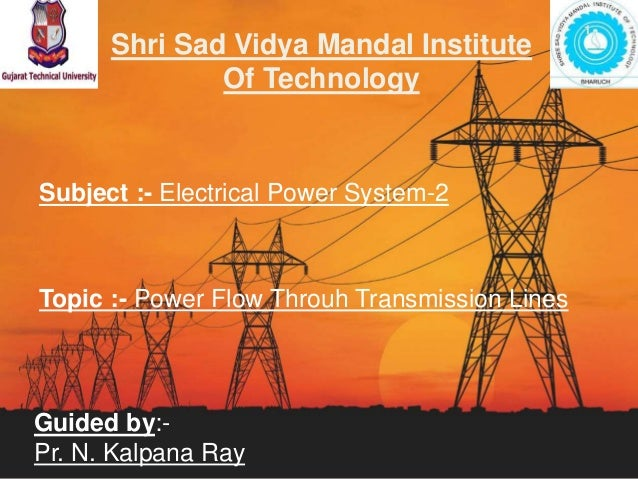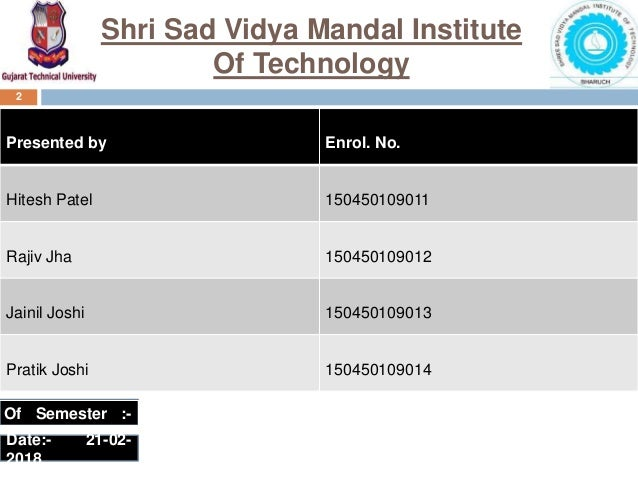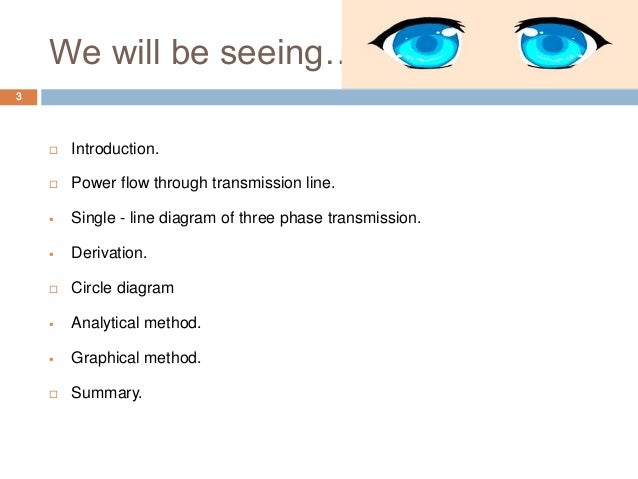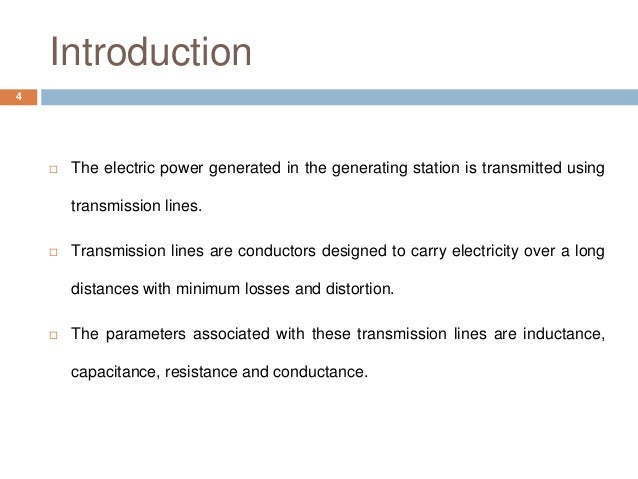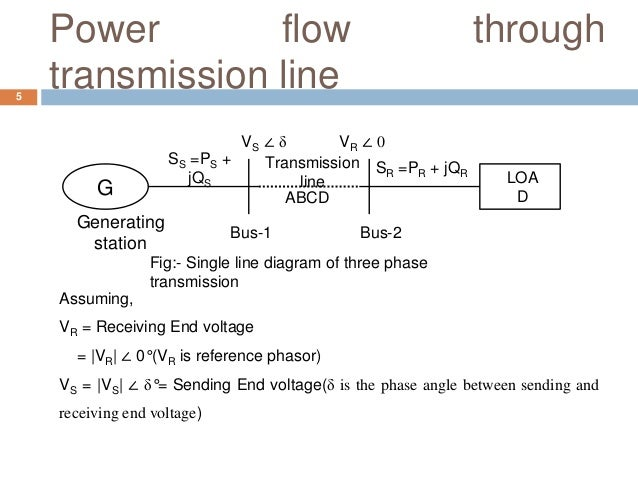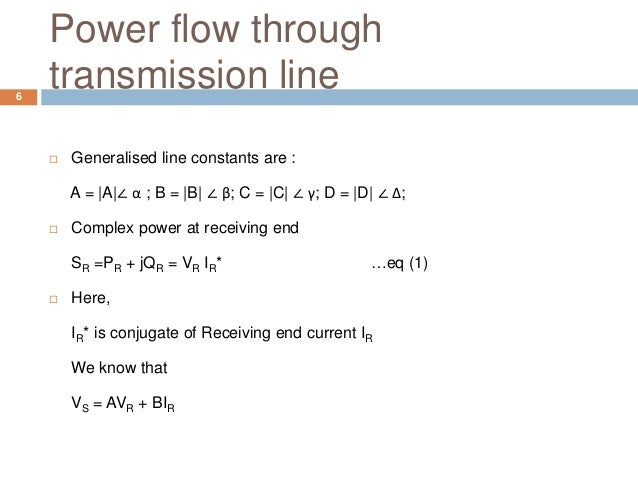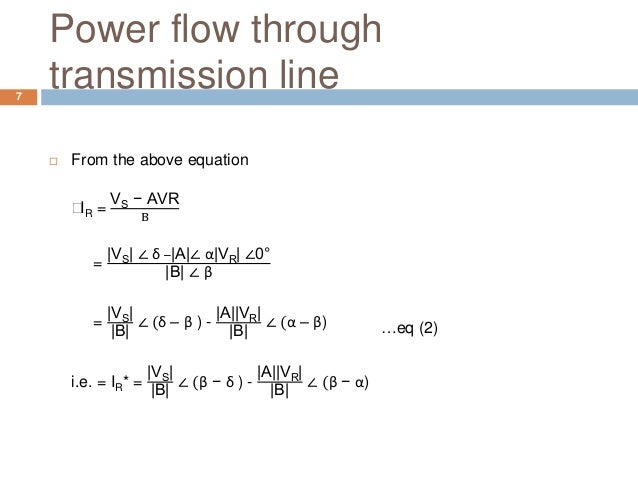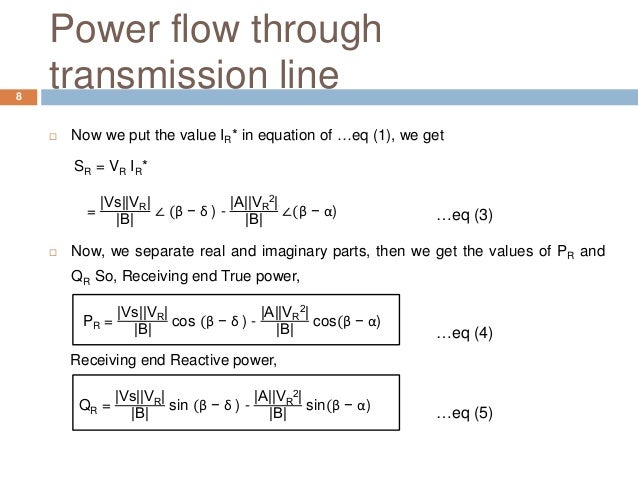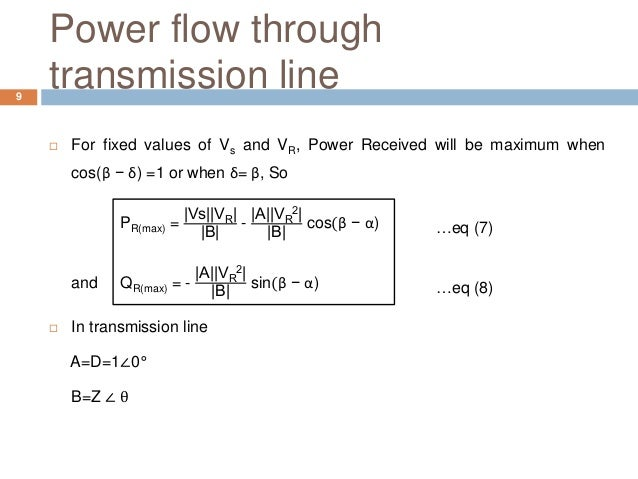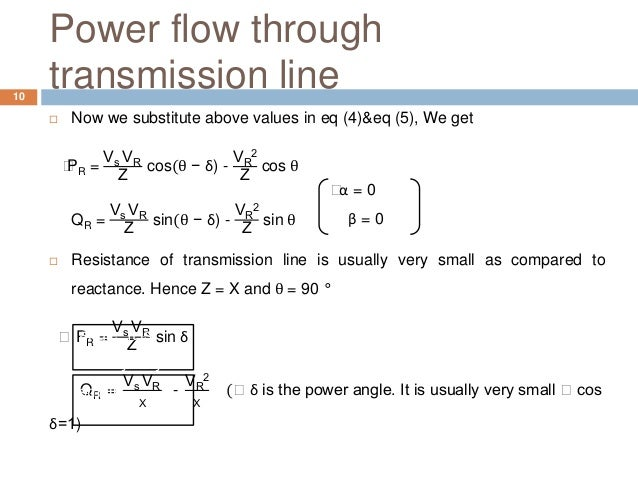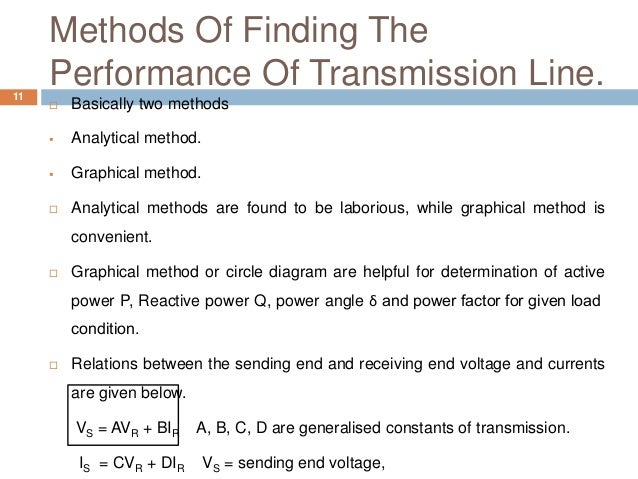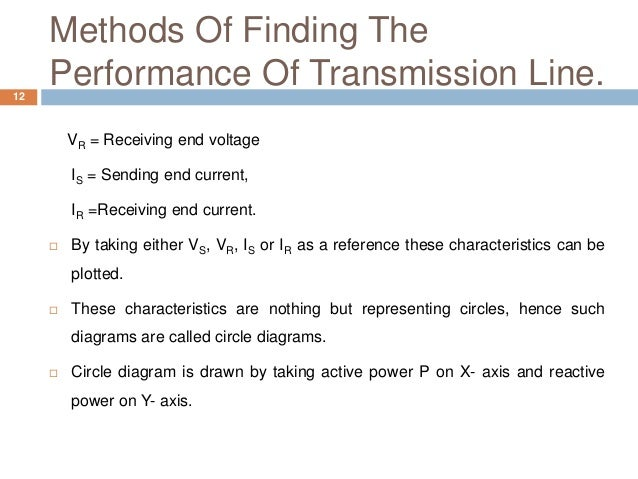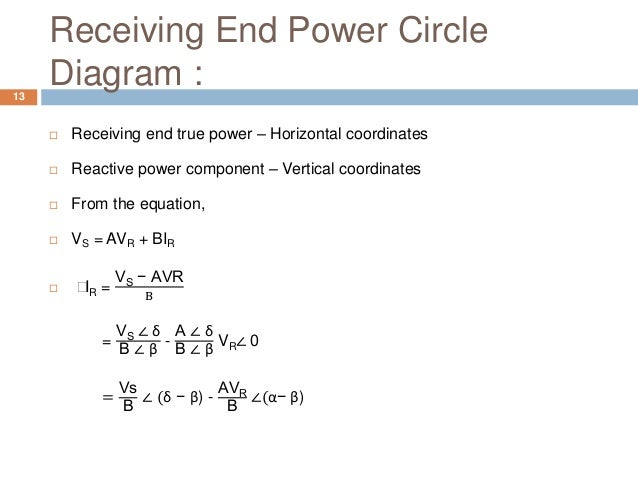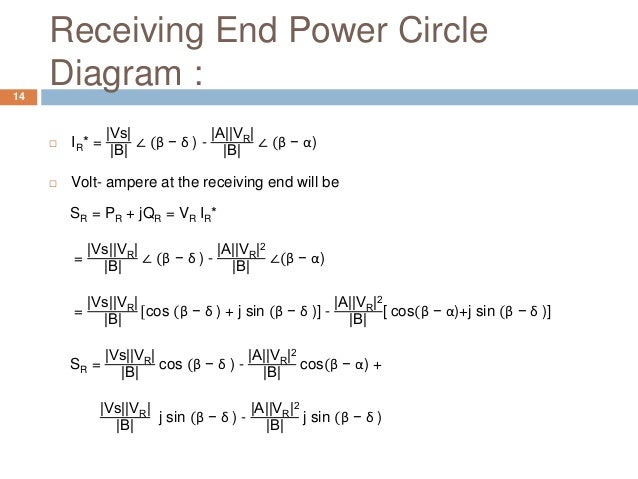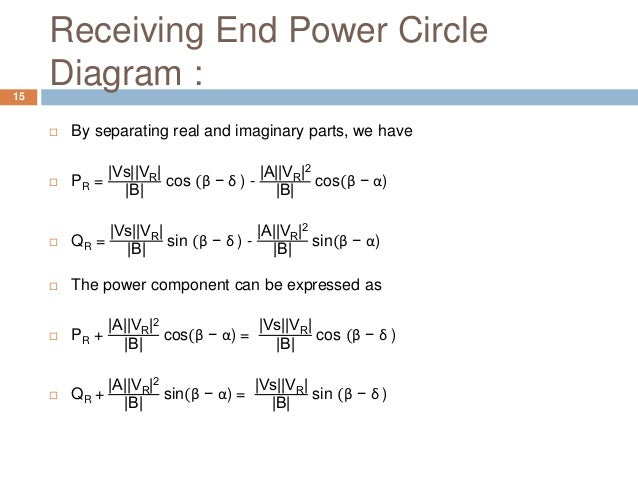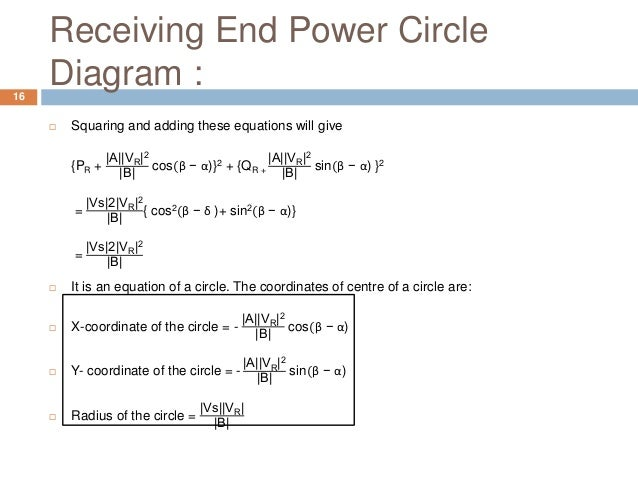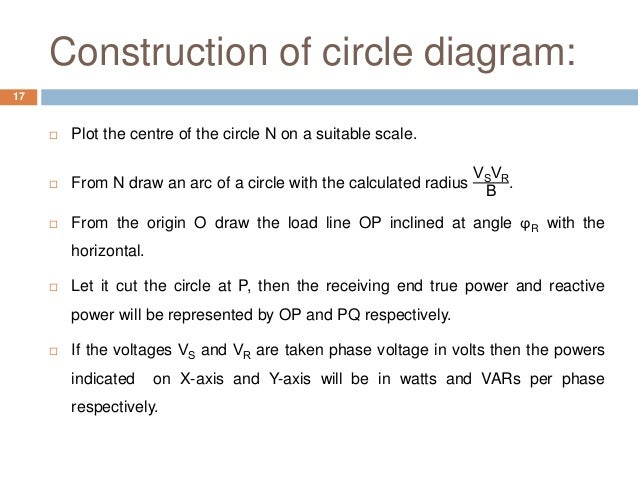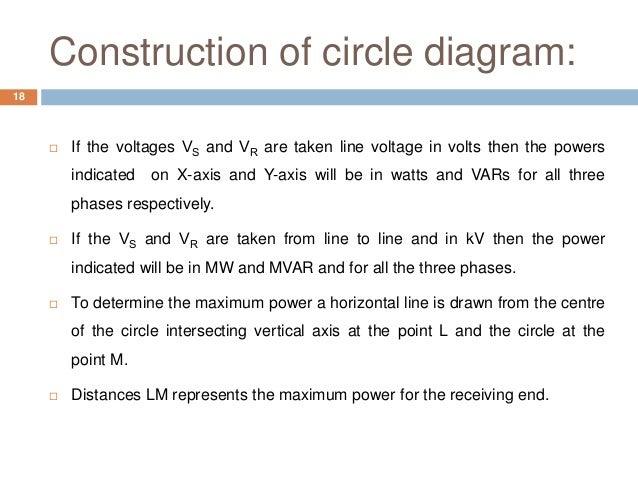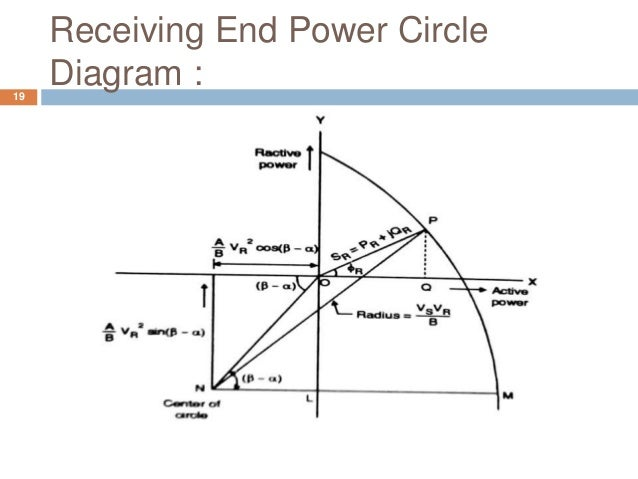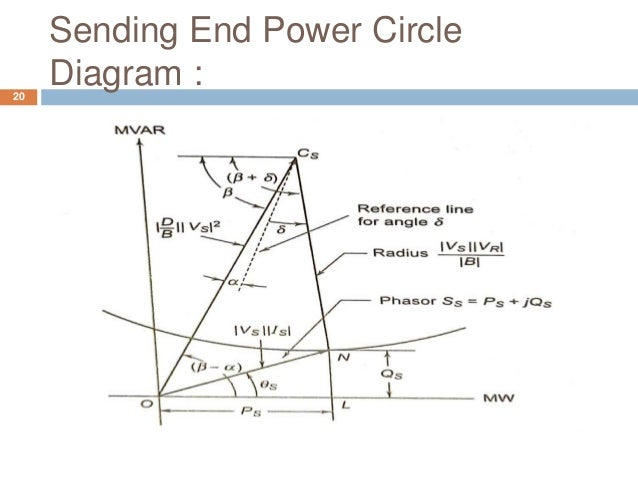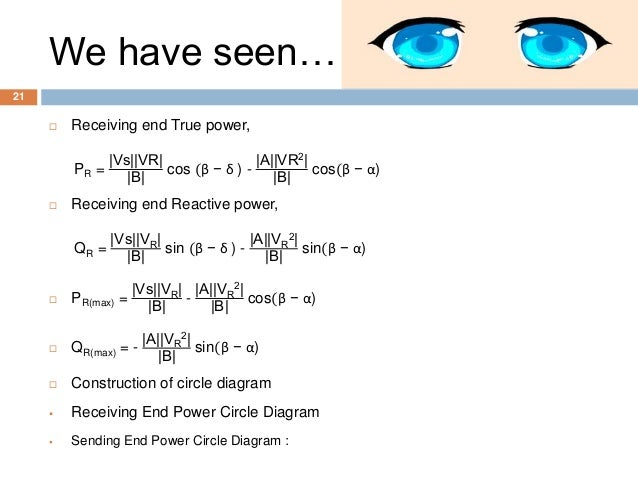Upcoming SlideShare
×
• Full Name
Comment goes here.

Are you sure you want to Yes No• Be the first to comment

### Power flow through transmission line.

1. 1. Shri Sad Vidya Mandal Institute Of Technology Subject :- Electrical Power System-2 Topic :- Power Flow Throuh Transmission Lines Guided by:- Pr. N. Kalpana Ray 1
2. 2. Of Semester :- Ⅵ 2 Presented by Enrol. No. Hitesh Patel 150450109011 Rajiv Jha 150450109012 Jainil Joshi 150450109013 Pratik Joshi 150450109014 Shri Sad Vidya Mandal Institute Of Technology Date:- 21-02- 2018
3. 3. We will be seeing…  Introduction.  Power flow through transmission line.  Single - line diagram of three phase transmission.  Derivation.  Circle diagram  Analytical method.  Graphical method.  Summary. 3
4. 4. Introduction  The electric power generated in the generating station is transmitted using transmission lines.  Transmission lines are conductors designed to carry electricity over a long distances with minimum losses and distortion.  The parameters associated with these transmission lines are inductance, capacitance, resistance and conductance. 4
5. 5. Power flow through transmission line G Generating station VS ∠ δ VR ∠ 0 SS =PS + jQS SR =PR + jQR LOA D Transmission line ABCD Bus-1 Bus-2 Fig:- Single line diagram of three phase transmission Assuming, VR = Receiving End voltage = |VR| ∠ 0°(VR is reference phasor) VS = |VS| ∠ δ°= Sending End voltage(δ is the phase angle between sending and receiving end voltage) 5
6. 6. Power flow through transmission line  Generalised line constants are : A = |A|∠ α ; B = |B| ∠ β; C = |C| ∠ γ; D = |D| ∠ Δ;  Complex power at receiving end SR =PR + jQR = VR IR*  Here, IR* is conjugate of Receiving end current IR We know that VS = AVR + BIR …eq (1) 6
7. 7. Power flow through transmission line  From the above equation ⸫IR = VS − AVR B = |VS| ∠ δ −|A|∠ α|VR| ∠0° |B| ∠ β = |VS| |B| ∠ (δ – β ) - |A||VR| |B| ∠ (α – β) i.e. = IR* = |VS| |B| ∠ (β − δ ) - |A||VR| |B| ∠ (β − α) …eq (2) 7
8. 8. Power flow through transmission line  Now we put the value IR* in equation of …eq (1), we get SR = VR IR* = |Vs||VR| |B| ∠ (β − δ ) - |A||VR 2| |B| ∠(β − α)  Now, we separate real and imaginary parts, then we get the values of PR and QR So, Receiving end True power, PR = |Vs||VR| |B| cos (β − δ ) - |A||VR 2| |B| cos(β − α) Receiving end Reactive power, QR = |Vs||VR| |B| sin (β − δ ) - |A||VR 2| |B| sin(β − α) …eq (4) …eq (3) …eq (5) 8
9. 9. Power flow through transmission line  For fixed values of Vs and VR, Power Received will be maximum when cos(β − δ) =1 or when δ= β, So PR(max) = |Vs||VR| |B| - |A||VR 2| |B| cos(β − α) and QR(max) = - |A||VR 2| |B| sin(β − α)  In transmission line A=D=1∠0° B=Z ∠ θ …eq (8) …eq (7) 9
10. 10. Power flow through transmission line  Now we substitute above values in eq (4)&eq (5), We get ⸫PR = Vs VR Z cos(θ − δ) - VR 2 Z cos θ QR = Vs VR Z sin(θ − δ) - VR 2 Z sin θ  Resistance of transmission line is usually very small as compared to reactance. Hence Z = X and θ = 90 ° ⸫ PR = Vs VR Z sin δ QR = Vs VR X - VR 2 X (⸫ δ is the power angle. It is usually very small ⸫ cos δ=1) ⸫α = 0 β = 0 δ is the power angle. It is usually very small 10
11. 11. Methods Of Finding The Performance Of Transmission Line.11  Basically two methods  Analytical method.  Graphical method.  Analytical methods are found to be laborious, while graphical method is convenient.  Graphical method or circle diagram are helpful for determination of active power P, Reactive power Q, power angle δ and power factor for given load condition.  Relations between the sending end and receiving end voltage and currents are given below. VS = AVR + BIR A, B, C, D are generalised constants of transmission. IS = CVR + DIR VS = sending end voltage,
12. 12. Methods Of Finding The Performance Of Transmission Line.12 VR = Receiving end voltage IS = Sending end current, IR =Receiving end current.  By taking either VS, VR, IS or IR as a reference these characteristics can be plotted.  These characteristics are nothing but representing circles, hence such diagrams are called circle diagrams.  Circle diagram is drawn by taking active power P on X- axis and reactive power on Y- axis.
13. 13. Receiving End Power Circle Diagram :13  Receiving end true power – Horizontal coordinates  Reactive power component – Vertical coordinates  From the equation,  VS = AVR + BIR  ⸫IR = VS − AVR B = VS ∠ δ B ∠ β - A ∠ δ B ∠ β VR∠ 0 = Vs B ∠ (δ − β) - AVR B ∠(α− β)
14. 14. Receiving End Power Circle Diagram :14  IR* = |Vs| |B| ∠ (β − δ ) - |A||VR| |B| ∠ (β − α)  Volt- ampere at the receiving end will be SR = PR + jQR = VR IR* = |Vs||VR| |B| ∠ (β − δ ) - |A||VR|2 |B| ∠(β − α) = |Vs||VR| |B| [cos (β − δ ) + j sin (β − δ )] - |A||VR|2 |B| [ cos(β − α)+j sin (β − δ )] SR = |Vs||VR| |B| cos (β − δ ) - |A||VR|2 |B| cos(β − α) + |Vs||VR| |B| j sin (β − δ ) - |A||VR|2 |B| j sin (β − δ )
15. 15. Receiving End Power Circle Diagram :15  By separating real and imaginary parts, we have  PR = |Vs||VR| |B| cos (β − δ ) - |A||VR|2 |B| cos(β − α)  QR = |Vs||VR| |B| sin (β − δ ) - |A||VR|2 |B| sin(β − α)  The power component can be expressed as  PR + |A||VR|2 |B| cos(β − α) = |Vs||VR| |B| cos (β − δ )  QR + |A||VR|2 |B| sin(β − α) = |Vs||VR| |B| sin (β − δ )
16. 16. Receiving End Power Circle Diagram :16  Squaring and adding these equations will give {PR + |A||VR|2 |B| cos(β − α)}2 + {QR + |A||VR|2 |B| sin(β − α) }2 = |Vs|2|VR|2 |B| { cos2(β − δ )+ sin2(β − α)} = |Vs|2|VR|2 |B|  It is an equation of a circle. The coordinates of centre of a circle are:  X-coordinate of the circle = - |A||VR|2 |B| cos(β − α)  Y- coordinate of the circle = - |A||VR|2 |B| sin(β − α)  Radius of the circle = |Vs||VR| |B|
17. 17. Construction of circle diagram: 17  Plot the centre of the circle N on a suitable scale.  From N draw an arc of a circle with the calculated radius VSVR B .  From the origin O draw the load line OP inclined at angle ϕR with the horizontal.  Let it cut the circle at P, then the receiving end true power and reactive power will be represented by OP and PQ respectively.  If the voltages VS and VR are taken phase voltage in volts then the powers indicated on X-axis and Y-axis will be in watts and VARs per phase respectively.
18. 18. Construction of circle diagram: 18  If the voltages VS and VR are taken line voltage in volts then the powers indicated on X-axis and Y-axis will be in watts and VARs for all three phases respectively.  If the VS and VR are taken from line to line and in kV then the power indicated will be in MW and MVAR and for all the three phases.  To determine the maximum power a horizontal line is drawn from the centre of the circle intersecting vertical axis at the point L and the circle at the point M.  Distances LM represents the maximum power for the receiving end.
19. 19. Receiving End Power Circle Diagram :19
20. 20. Sending End Power Circle Diagram :20
21. 21. We have seen…  Receiving end True power, PR = |Vs||VR| |B| cos (β − δ ) - |A||VR2| |B| cos(β − α)  Receiving end Reactive power, QR = |Vs||VR| |B| sin (β − δ ) - |A||VR 2| |B| sin(β − α)  PR(max) = |Vs||VR| |B| - |A||VR 2| |B| cos(β − α)  QR(max) = - |A||VR 2| |B| sin(β − α)  Construction of circle diagram  Receiving End Power Circle Diagram  Sending End Power Circle Diagram : 21

### Be the first to comment

•May. 10, 2018
•#### ssuser508167

Jul. 24, 2018
•#### kgprajapati

Jan. 2, 2020
•#### SandiAungMin

Mar. 1, 2020
•#### dhruvrajraulji1

Apr. 20, 2020
•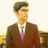#### 722446

Apr. 30, 2020
•#### HayelZayed

Dec. 5, 2020
•#### PraveenLucky8

Mar. 6, 2021
•#### NtemiM

Jun. 29, 2021

Total views

6,853

On Slideshare

0

From embeds

0

Number of embeds

5

171

Shares

0